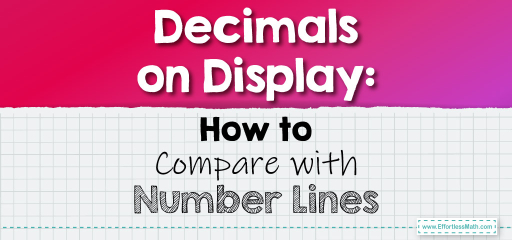# Decimals on Display: How to Compare with Number Lines

Number lines are powerful tools for visualizing and comparing decimals. By placing decimals on a number line, we can easily see their relative positions and determine which one is greater, smaller, or if they are equal. Let's explore how to use number lines for comparing decimals.## Comparing Decimals Using Number Lines

### Example 1:

Decimals to Compare: $$0.4$$ and $$0.35$$

Using a Number Line:

Draw a number line from $$0$$ to $$1$$. Mark the positions of $$0.4$$ and $$0.35$$ on the line.

By observing their positions, we can see that $$0.4$$ is to the right of $$0.35$$, indicating that $$0.4$$ is greater.

$$0.4 > 0.35$$

The Absolute Best Book for 5th Grade Students

### Example 2:

Decimals to Compare: $$0.65$$ and $$0.7$$

Using a Number Line:

Draw a number line from $$0$$ to $$1$$. Mark the positions of $$0.65$$ and $$0.7$$ on the line.

By observing their positions, $$0.7$$ is to the right of $$0.65$$, indicating that $$0.7$$ is greater.

$$0.7 > 0.65$$

Number lines provide a clear visual representation of decimals, making it easier to compare them. This method is especially beneficial for those who prefer visual learning. By practicing with number lines, you’ll gain a deeper understanding of the relative values of decimals and enhance your comparison skills!

### Practice Questions:

1. Place $$0.5$$ and $$0.55$$ on a number line. Which is greater?

2. Compare $$0.72$$ and $$0.8$$ using a number line. Which is smaller?

3. Determine the larger decimal between $$0.3$$ and $$0.29$$ using a number line.

4. Which is smaller when placed on a number line: $$0.9$$ or $$0.91$$?

5. Compare $$0.123$$ and $$0.13$$ on a number line. Which is greater?

A Perfect Book for Grade 5 Math Word Problems!

1. $$0.55$$

2. $$0.72$$

3. $$0.3$$

4. $$0.9$$

5. $$0.13$$

The Best Math Books for Elementary Students

### What people say about "Decimals on Display: How to Compare with Number Lines - Effortless Math: We Help Students Learn to LOVE Mathematics"?

No one replied yet.

X
51% OFF

Limited time only!

Save Over 51%

SAVE $15 It was$29.99 now it is \$14.99